Next: Pulse trains via wavetable Up: Pulse trains Previous: Pulse trains   Contents   Index

## Pulse trains via waveshaping

When we use waveshaping the shape of the formant is determined by a modulation termFor small values of the index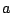, the modulation term varies only slightly from the constant value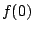, so most of the energy is concentrated at DC. Asincreases, the energy spreads out among progressively higher harmonics of the fundamental. Depending on the function, this spread may be orderly or disorderly. An orderly spread may be desirable and then again may not, depending on whether our goal is a predictable spectrum or a wide range of different (and perhaps hard-to-predict) spectra.

The waveshaping function, analyzed on Page, gives well-behaved, simple and predictable results. After normalizing suitably, we got the spectra shown in Figure 5.13. A slight rewriting of the waveshaping modulator for this choice of(and taking the renormalization into account) gives: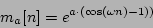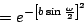where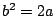so thatis proportional to the bandwidth. This can be rewritten as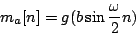withExcept for a missing normalization factor, this is a Gaussian distribution, sometimes called a bell curve". The amplitudes of the harmonics are given by Bessel I" type functions.

Another fine choice is the (again unnormalized) Cauchy distribution:which gives rise to a spectrum of exponentially falling harmonics:whereand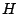are functions of the index(explicit formulas are given in [Puc95a]).

In both this and the Gaussian case above, the bandwidth (counted in peaks, i.e., units of) is roughly proportional to the index, and the amplitude of the DC term (the apex of the spectrum) is roughly proportional to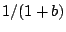. For either waveshaping function (or), ifis larger than about 2, the waveshape ofis approximately a (forward or backward) scan of the transfer function, so the resulting waveform looks like pulses whose widths decrease as the specified bandwidth increases.Next: Pulse trains via wavetable Up: Pulse trains Previous: Pulse trains   Contents   Index
Miller Puckette 2006-12-30